# Scratch 1.4

Version of implementation Scratch of programming language Scratch

A version of Scratch IDE.

## Examples:

### Hello, World! - Scratch (129):

Since it’s a graphical language, there is no actual source text, see the print-screen instead. The bat is the sprite of the example, and the script which is associated with it has only one block which makes the sprite “say” the required phrase.

``````say Hello, World!
``````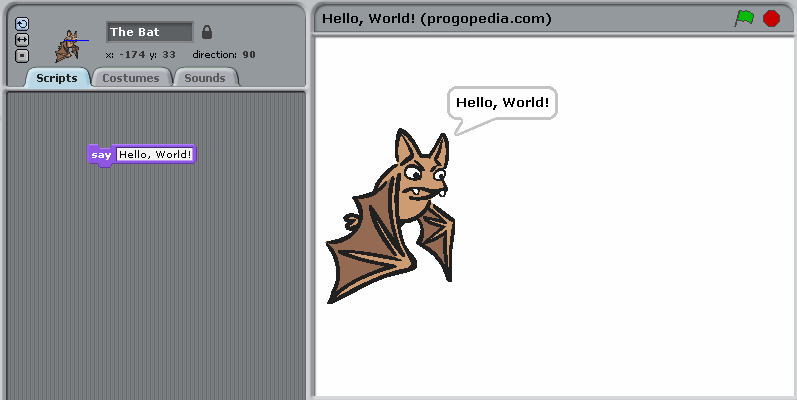"Hello, World!" example in Scratch

### Factorial - Scratch (130):

Since Scratch is a graphical language, the print-screen contains the actual information about the program, source text is only a transcription.

`strs` is a vector-style array. `delete all` block is required to clean it before running program again. `repeat` is a kind of loop which repeats its body for exactly the given number of times. `join` is a block which concatenates its arguments. `hide` hides the avatar (to clean the stage).

Scratch doesn’t have a standard output as such, neither has it a possibility of “saying” multi-line things. An array was used to output the example results in the required form.

``````delete all of strs
set i to 0
set f to 1
repeat 17
add (join i (join (! = ) f)) to strs
set i to (i + 1)
set f to (f * i)
hide
``````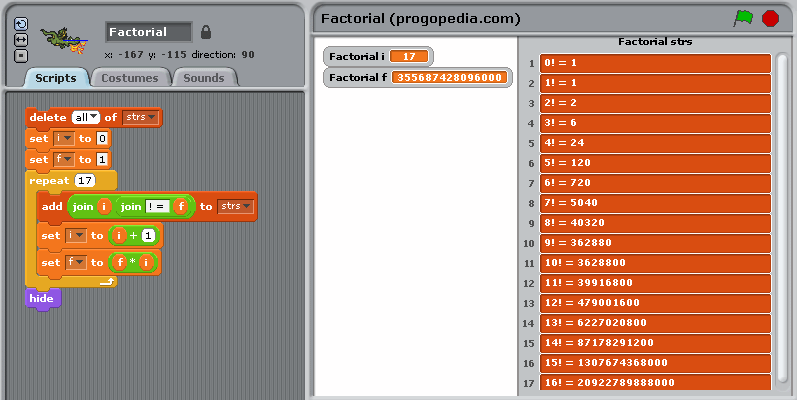Factorial example in Scratch

### Fibonacci numbers - Scratch (131):

This example uses recursive definition of Fibonacci numbers, since Scratch doesn’t have an easy way to define a function. Besides, Scratch is a graphical language, the print-screen contains the actual information about the program, source text is only a transcription.

``````set f1 to 1
set f2 to 1
set str to f1
repeat 15
set f3 to (f1 + f2)
set f1 to f2
set f2 to f3
set str to join (str (join (,) f1))
say join (str (...))
``````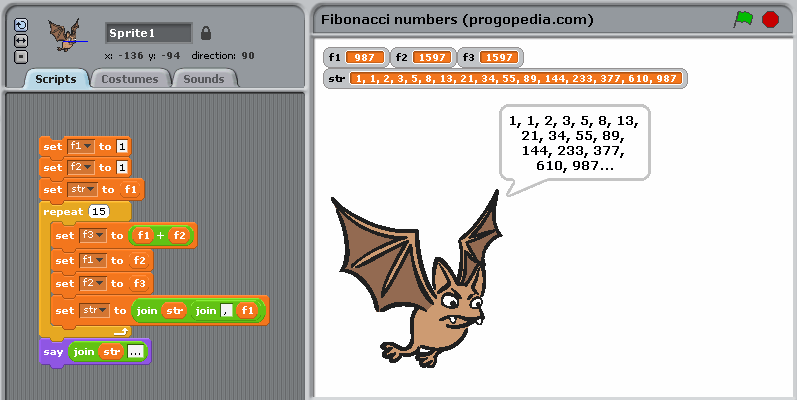Fibonacci numbers example in Scratch

### Quadratic equation - Scratch (175):

Scratch is a graphical language, the print-screen contains the actual information about the program, source text is only a transcription. This example features the usage of text input in Scratch, which is done with command `ask "..." and wait`. This command makes the sprite “say” the associated message (used as prompt) and displays an input window below the sprite. Once the information is entered, is can be accessed through predefined variable `answer`, which is copied to local variables before entering next values.

``````ask "A = ?" and wait
set A to answer
if A = 0
say "Not a quadratic equation"
else
ask "B = ?" and wait
set B to answer
ask "C = ?" and wait
set C to answer
set D to B*B + (-4)*A*C
set re to B/(-2*A)
if D = 0
say join ("x = " re)
else
set im to (sqrt of (abs of D)) / (2*A)
if D > 0
say join (join ("x1 = " (re+im)) join (", x2 = " (re-im)))
else
say join (join ("x1 = (" join (re join (", " im))) join ("), x2 = (" join (re join (", -" join (im ")")))))
``````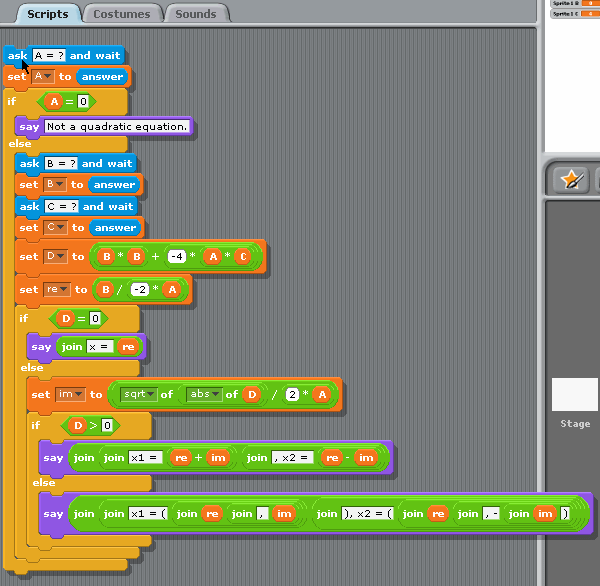Quadratic equation example in Scratch

### CamelCase - Scratch (299):

Since Scratch is a graphical language, the screenshot contains all the actual information about the program.

This example can’t be done using only standard blocks provided by the language; to implement it, we’ll need to explore hidden possibilities of the environment. Two blocks which handle conversions between ASCII codes and letters can be added as described in this tutorial on Scratch web-site. Once they are available, the rest of the program is quite evident.

Note that arrays are indexed starting with 1, and boolean values have to be compared with `true` and `false` explicitly to produce a condition.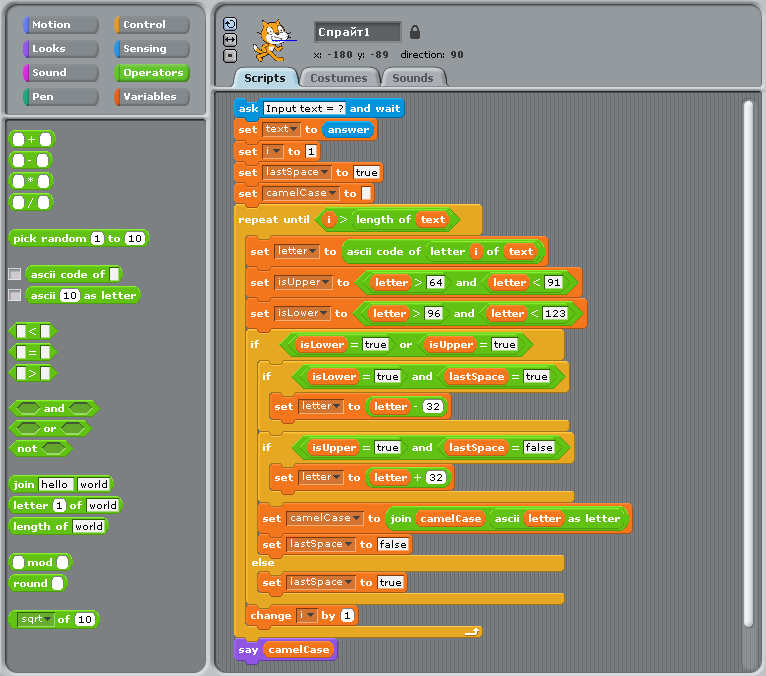CamelCase example in Scratch